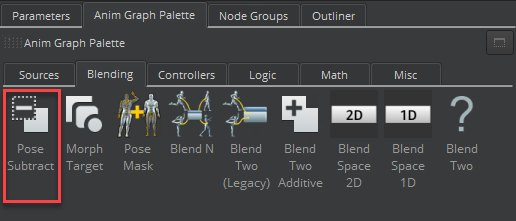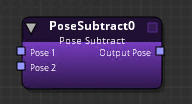Version:

# Pose Subtract Node

The Pose Subtract node subtracts Pose 2 from Pose 1. The output of a Pose Subtract node is the delta between them (Pose 1 - Pose 2).

Note:
With the Pose Subtract node, you can generate an additive pose. You can then supply it to the Blend Two Additive node at runtime without manually generating it from a DCC. The output of the Pose Subtract node cannot be used as an input to the Blend Two (Legacy) node. This is because Blend Two (Legacy) does not expect a pose that is already a delta or additive pose, but expects a bind pose.

To use the Pose Subtract node

1. In O3DE Editor, choose Tools, Animation Editor.

2. Create a blend tree.

3. Double-click the blend tree node that you created.

4. Select the Anim Graph Palette tab and then select the Blending tab.

5. Drag the Pose Subtract node into the animation graph.Connect nodes to the following inputs and output:

• Pose 1 - The base pose.
• Pose 2 - The pose to be subtracted from the base pose.
• Output Pose - The result of the pose subtraction (Pose 1 - Pose 2).

## Pose Subtract Node Attributes

For attribute settings that are shared among the blend node types, see Blend Node Attributes.